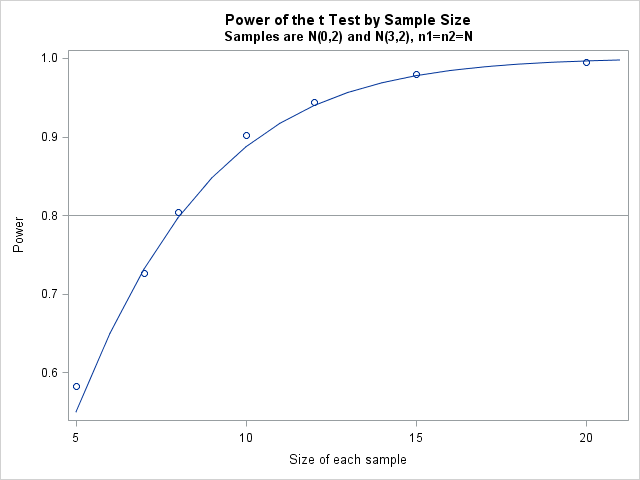## T-Test simulation

Hello,

I have posted a question on the macro and DATA step forum, which is related do simulations, and more precisely to a simulation from Rick Wicklin's book, so I was advised to post it here although it's not really about IML or matrices.

I bought the "Simulating Data with SAS book". I am relatively new to SAS programming (although was using SAS for analysis for some time), and wishes to improve my SAS skills, in particular the ones required for the clinical world.

I read the program in section 5.4.2 about simulating the power of the t-test. The program assumes varying effect sizes. I wanted to change the code so it will suit varying sample sizes rater than effect sizes (it would be ideal if both could vary but this is complicated).

After fixing my code several times because I got it wrong, I finally got results which are almost identical to the ones of the power proc. However, in page 86 in the book there is a graph. When I ran Rick's code, I got this graph too. After I changed the program, the line disappeared, only the dots were presented. When I changed the series x=N y=p, I got a line, that connects my points, but it wasn't the line of proc power. When I keep it as series x=N y=Power, I get dots only. I can't figure out why, I tried comparing the output files, the structure looked identical.

My next stage will be to try and simulate power for other statistical tests and models, I will try and most likely ask a few questions in the future. My ideal goal will be to create a small library of power analysis code for several situations (superiority, non-inferiority, equivalence) of different variables (continuous, binary, time to event, ...).

Thank you !

proc power;

twosamplemeans  power = .

meandiff = 3

stddev = 2

ntotal=10 to 40 by 10;

plot x=N markers=none;

ods output Output=Power;

run;

data PowerSim(drop=i);

call streaminit(321);

do N = 5 to 20 by 5;

do SampleID = 1 to 10000;

c = 1;

do i = 1 to N;

x1 = rand("Normal", 0, 2);

output;

end;

c = 2;

do i = 1 to N;

x1 = rand("Normal", 3, 2);

output;

end;

end;

end;

run;

proc ttest data=PowerSim;

by N SampleID;

class c;

var x1;

ods output ttests=TTests(where=(method="Pooled"));

run;

data Results;

set TTests;

RejectH0 = (Probt le 0.05);

run;

proc freq data=Results noprint;

by N;

tables RejectH0 / out=SimPower(where=(RejectH0=1));

run;

data Combine;

set SimPower Power;

p = percent / 100;

label p="Power";

run;

proc sgplot data=Combine noautolegend;

title "Power of the t Test";

title2 "Samples are N(0,stdev) and N(delta,stdev)";

series x=N y=Power;

scatter x=N y=p;

xaxis label="Sample size";

run;

1 ACCEPTED SOLUTION

Accepted Solutions

## Re: T-Test simulation

It sounds like you want to use the code provided in Section 5.5 to produce a graph similar to Figure 5.10.

However, you deleted several important lines of code, including suppressing the output from PROC TTEST.

In particular you deleted the statement

NSamp = NTotal / 2;

in the DATA COMBINE step.  The call to PROC SGPLOT needs to use the NSamp variable for the SERIES statement and the N variable for the SCATTER statement.

I've attached the program that I think you are trying to create.3 REPLIES 3

## Re: T-Test simulation

It sounds like you want to use the code provided in Section 5.5 to produce a graph similar to Figure 5.10.

However, you deleted several important lines of code, including suppressing the output from PROC TTEST.

In particular you deleted the statement

NSamp = NTotal / 2;

in the DATA COMBINE step.  The call to PROC SGPLOT needs to use the NSamp variable for the SERIES statement and the N variable for the SCATTER statement.

I've attached the program that I think you are trying to create.## Re: T-Test simulation

Thank you very much, I appreciate it.

I did suppressing the output, I just didn't copy this part here, assuming that the problem wasn't there. I think I understand what went wrong.

If I want to convert your code to a case of a non-inferiority hypothesis, will the only thing I'll have to do be to change the t-test to a one sided test compared to a non zero number ?

## Re: T-Test simulation

I'm not sure, but I would try to get PROC TTEST to do most of the work. The SIDES= and H0= options enable you to specify whatever null hypothesis you are interested in.  See

the 2015 SAS Global Forum paper by Castello and Watts: http://support.sas.com/resources/papers/proceedings15/SAS1911-2015.pdf

Message was edited by: Rick Wicklin

From The DO Loop